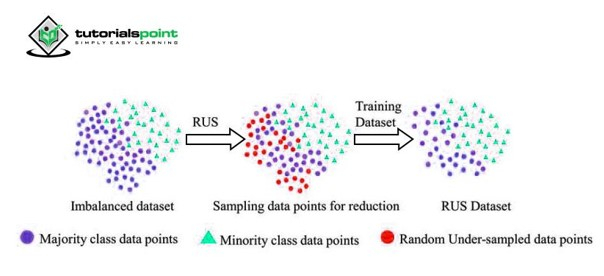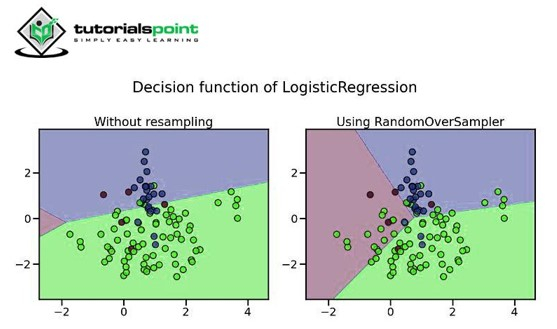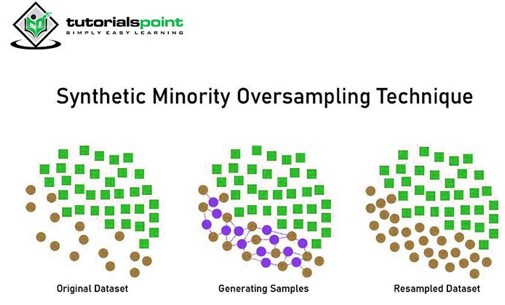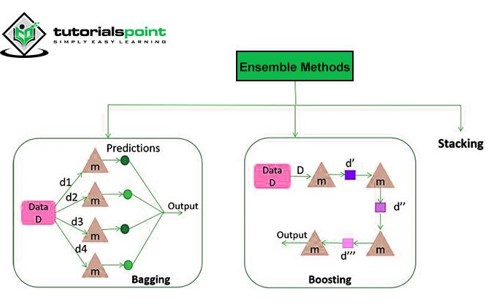# How to Handle Imbalanced Classes in Machine Learning

In this tutorial, we are going to learn about how to handle imbalanced classes in ML.

## Introduction

Generally speaking, class imbalance in Machine Learning is a case where classes of one type or observation are higher as compared to the other type. It is a common problem in Machine learning involving tasks such as fraud detection, ad click averts, spam detection, consumer churn, etc. It has a high effect on the accuracy of the model.

## Effects of Class Imbalance

In case of such problems, the majority class overpowers the minority class while training the model. Since in such cases, one type of class is very high in number compared to the other (generally < 0.05%) the model trained will mostly predict the majority class. This adversely affects the accuracy and the results are prone to errors.

So, how can we deal with this problem? Let's dive into a few of these techniques that have proved beneficial.

## How to deal with class imbalance

There are 5 such methods employed to deal with the imbalance class problems.

### 1. Random Undersampling

In Credit Card fraud detection systems, we mostly face problems of class imbalance since the number of fraudulent transactions is significantly low ( sometimes less than 1%). Random under-sampling is one such simple technique to deal with the problem. In this method, the data points from the majority class are removed to a significant level so that it balances out the minority class. The majority and minority classes are in comparable ratiosIt is useful when we have a lot of data.

One disadvantage of this method is that it can lead to major information loss and can produce a less accurate model.

### 2. Random Oversampling

In this method, the minority class is replicated multiple times in a fixed integer ratio so that it balances out the majority class. We should remember to use K-Fold cross-validation before oversampling so that appropriate randomness can be introduced in the data to prevent overfitting.This method is very useful when we have fewer data.

The drawback of this method is that it may lead to overfitting and the produced model will not generalize well.

### 3. Synthetic Minority Oversampling Technique (SMOTE)

SMOTE is a statistical technique to increase the sample in minority classes in a balanced way. It generates new instances from the existing minority classes. It does not affect the majority class. This algorithm takes samples from the target class and its k-nearest neighbors. Then it creates samples by combing features from the target class and its neighbors.The advantage of SMOTE is that we are not just replicating the minority class but creating synthetic data points that are different from the original ones

There are a few disadvantages of SMOTE

• It is difficult to determine the number of nearest neighbors and the selection of nearest neighbors is not accurate.

• It might oversample noisy samples

### 4. Ensemble Learning

In this method, we use multiple learners or combine the results of multiple classifiers to produce the desired result. It is proven that many a time multiple learners may produce better results than a single one.Two methods involved are Bagging and Boosting

• Bagging

The bagging method is known as bootstrapped aggregation. Bootstrapping is the method of creating samples of data from the population to estimate a population parameter. In Bagging small classifiers are trained on such samples of data taken from the dataset with replacements. The prediction of these classifiers is then aggregated to produce the final result.

•     One advantage of bagging is that it reduces the variance error of the models.

• Boosting

It is a method to reduce errors in prediction by adjusting the weights of observation based on the most recent classification. It tends to increase the weights of the observation which was incorrectly classified. This model is trained on the errors produced by the previous models. This iterative approach is highly parallelable.

•     One advantage of boosting is that it reduces the bias error of the models.

## Choosing the right evaluation metric.

Choosing accuracy as the evaluation parameter for datasets with class imbalance may prove disastrous. A better metric is precision or recall or a combined metric such as F-score. while the accuracy metric seems to get adversely affected due to improper proportions of classes, precision/recall/F1-score seems to handle this problem well as evident below from their relations

$$\mathrm{Precision \:=\: \frac{True\: Positives}{True\: Positives\:+\:False\: Positives}}$$ $$\mathrm{Recall \:=\: \frac{True\: Positives}{True\: Positives\:+\:False\: Positives}}$$

F1 scores consider how many correct classifications were made

$$\mathrm{F1\:-\:Score \:=\: \frac{2\:*\:Precision\:*\:Recall}{Precision\:+\:Recall}}$$

## Conclusion

Thus, we saw how class imbalance can seriously affect our predictions based on model performance. Handling such issues may sometimes prove tedious, but as we saw in this article, the right technology combined with proper evaluation metrics can solve this issue, thereby increasing the quality of our models' predictions.

Updated on: 01-Dec-2022

238 Views